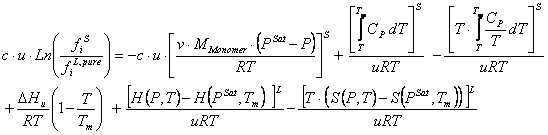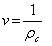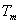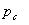﻿ Solid-liquid calculations (SLE)

# Solid-liquid calculations (SLE)

Top  Previous  Next

Solid-liquid calculations (SLE) are of equal importance as that the liquid-vapor calculations (VLE) are, since during the production of polymers solid polymer is the end product and is often not desired to occur during the process in order to prevent clogging of the equipment.

When performing SLE calculations the thermodynamic model is not the same for the different phases. Liquid and vapor phases are modeled by using an equation of state (EOS) and the solid phase is modeled by using a separate model that can only be used for solid phases.

The approach taken in VLXE is taken from three articles given in the references below.

Two different versions of the same model is implemented:

The heat capacity terms are dropped and the volume for both solid and liquid is assumed to be constantWhere:The heat capacity terms are keep intact and the liquid phase is modeled by using the selected equation of state:where:User input:

 Parameter Description Value of polyethylene c Gives the fraction of the solid polymer i.e crystal Defined by the userEnthalpy of mixing per mole of crystal unit 8220 J/moleMelting temperature for each pseudo component Defined by the userDensity of amorphous polymer 0.853 g/cm3Density of crystalline polymer 1.004 g/cm3Molar mass of monomer 28.054 g/mole (Molar mass of ethylene)Solid->Solid transition temperature Defined by the userEnthalpy of Solid->Solid transition Defined by the userHeat capacity of solid polymer 4th order polynomial Defined by the user

The possible SLE calculations are:

Cloud temperature

Cloud polymer mass fraction

Flash at fixed temperature and pressure.

Cloud temperature line at fixed pressure.